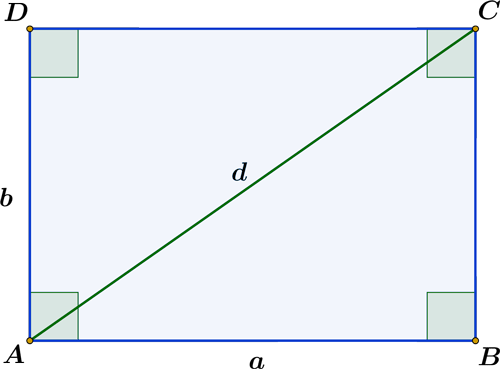Math Calculators, Lessons and Formulas

It is time to solve your math problem

mathportal.org

# Rectangle calculator

Select the missing value after entering two rectangle elements (length, width, diagonal, area, or perimeter). The calculator will provide you a step-by-step solution to the given problem.
show help ↓↓ examples ↓↓ tutorial ↓↓
Input what to find:
Input any two known values of a rectangle
calculator works with decimals, fractions and square roots ( to input $\color{blue}{\sqrt{2}}$ type $\color{blue}{\text{r2}}$)
$a$
=
$b$
=
$d$
=
AreaA
=
PerimP
=

working...
EXAMPLE PROBLEMS
example 1:ex 1:
Find the area of the rectangle whose sides are $a = \dfrac{5}{3}$ and $b = \dfrac{3}{2}$.
example 2:ex 2:
If the diagonal is 9 cm and one side is 5 cm, find the area of a rectangle.
example 3:ex 3:
A rectangle has an area of 18 cm2 and a side length of 16/5cm. Determine the perimeter.
Search our database of more than 200 calculators
TUTORIAL

## Rectangle calculations

This calculator uses the following formulas to find the missing values of a rectangle:

 Area: $$A = a \cdot b$$Perimeter: $$P = 2a + 2b$$ Diagonal: $$d^2 = a^2 + b^2$$

### Example 01 :

What is the area of a rectangle with a base of 12 cm and a height of 3/2 cm?

### Solution:

base $a = 6$

height $b = \dfrac{9}{2}$

$$\color{blue}{A = a \cdot b} = 6 \cdot \frac{9}{2} = \frac{54}{2} = 27$$

### Example 02 :

What is the perimeter of a rectangle with a length of 7/2cm and a width of 5/2cm?

### Solution:

length $a = \dfrac{7}{2} \, cm$

width $b = \dfrac{5}{2} \, cm$

$$\color{blue}{P = 2a + 2b} = 2 \cdot \frac{7}{2} + 2 \cdot \dfrac{5}{2} = 7 + 5 = 12$$

### Example 03 :

The area of a rectangle is 42 cm2. Find its perimeter if the width is 7cm.

### Solution:

We'll need two steps to solve this one:

Step 1: find length ( b ):

width $a = 7 cm$

area: $A = 42 cm$

\begin{aligned} A & = a \cdot b \\[ 1 em] 42 & = 7 \cdot b \\[ 1 em] b & = \frac{42}{7}\\[ 1 em] b & = 6 \\[ 1 em] \end{aligned}

Step 2: find perimeter ( P )

width $a = 7 cm$

length $b = 6 cm$

\begin{aligned} P & = 2a + 2b \\[ 1 em] P & = 2 \cdot 7 + 2 \cdot 6 \\[ 1 em] P & = 14 + 12 \\[ 1 em] P & = 28 \, cm^2 \\[ 1 em] \end{aligned}

### Example 04 :

What is the diagonal of a rectangle if the perimeter is P = 11/2 cm and a width is a = 3/2 cm ?

### Solution:

Step 1: find length ( b ):

width $a = \dfrac{3}{2} cm$

perimeter: $P = \dfrac{11}{2} cm$

\begin{aligned} P & = 2a + 2b \\[ 1 em] \frac{11}{2} & = 2 \cdot \frac{3}{2} + 2b \\[ 1 em] \frac{11}{2} & = 3 + 2b \\[ 1 em] 2b &= \frac{11}{2} - 3 \\[1 em] 2b &= \frac{5}{2} \\[1 em] b &= \frac{5}{4} \end{aligned}

Step 2: find diagonal ( d )

width $a = \dfrac{3}{2} cm$

length $b = \dfrac{5}{4} cm$

\begin{aligned} d^2 & = a^2 + b^2 \\[ 1 em] d^2 & = \left( \frac{3}{2} \right)^2 + \left( \frac{5}{4} \right)^2 \\[ 1 em] d^2 & = \frac{9}{4} + \frac{25}{16} \\[ 1 em] d^2 & = \frac{61}{16} \\[ 1 em] d & = \frac{\sqrt{61}}{4} \end{aligned}
Search our database of more than 200 calculators